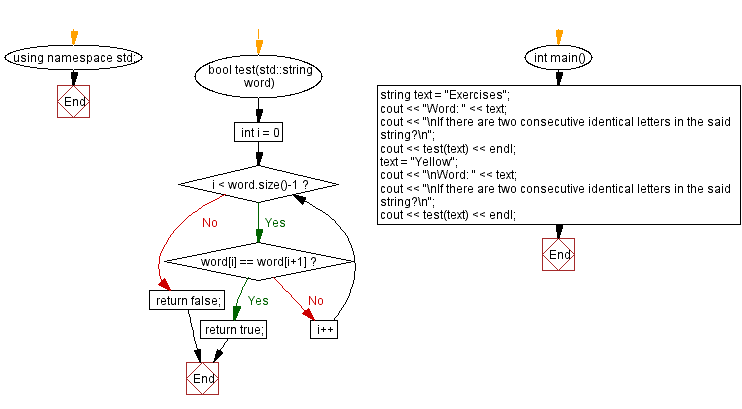﻿ C++ - Check two consecutive, identical letters in a word

# C++ String Exercises: Check two consecutive, identical letters in a word

## C++ String: Exercise-36 with Solution

Write a C++ program to check whether there are two consecutive (following each other continuously), identical letters in a given string.

Test Data:
("Exercises") -> 0
("Yellow") -> 1

Sample Solution:

C++ Code:

``````#include <bits/stdc++.h>

using namespace std;

bool test(std::string word) {
for(int i = 0; i < word.size()-1; i++){
if(word[i] == word[i+1]){
return true;
}
}
return false;
}
int main()
{
string text = "Exercises";
cout << "Word: " << text;
cout << "\nIf there are two consecutive identical letters in the said string?\n";
cout << test(text) << endl;
text = "Yellow";
cout << "\nWord: " << text;
cout << "\nIf there are two consecutive identical letters in the said string?\n";
cout << test(text) << endl;
}
``````

Sample Output:

```Word: Exercises
If there are two consecutive identical letters in the said string?
0

Word: Yellow
If there are two consecutive identical letters in the said string?
1

```

Flowchart:C++ Code Editor:

Previous C++ Exercise: Reverse the words in a string that have odd lengths.

Next C++ Exercise: Count occurrences of a certain character in a string.

What is the difficulty level of this exercise?

﻿

## C++ Programming: Tips of the Day

Advantage of switch over if-else statement

Use switch.

In the worst case the compiler will generate the same code as a if-else chain, so you don't lose anything. If in doubt put the most common cases first into the switch statement.

In the best case the optimizer may find a better way to generate the code. Common things a compiler does is to build a binary decision tree (saves compares and jumps in the average case) or simply build a jump-table (works without compares at all).

Ref: https://bit.ly/3G0uBqS

We are closing our Disqus commenting system for some maintenanace issues. You may write to us at reach[at]yahoo[dot]com or visit us at Facebook